### Lines And Angles Class 9th Mathematics AP Board Solution

##### Question 1.In the given figure three lines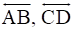and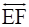intersecting at O. Find the values of x, y and z it is being given that x : y : z = 2 : 3 : 5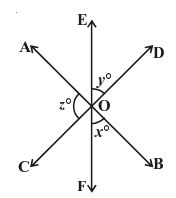Answer:From the given, the three angles are x,y,zIf the two lines intersect at a point then its vertically opposite angles are equal.∴ A = x, B = z and C = yWe know that,The sum of all the angles around at a point is equal to 360°∴ A + B + C + x + y + z = 360°⇒ x + y + z + x + y + z = 360°⇒ 2x + 2y + 2z = 360°⇒ 2(x + y + z) = 360°⇒ (x + y + z) =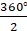⇒ x + y + z = 180° ------(1)Given that, x : y : z = 2 : 3 : 5Let x = 2m,y = 3m,z = 5m (∵ m = constant)Substitute these values in equation (1) we get2m + 3m + 5m = 18010m = 180m =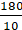∴ m = 18Substituting m = 18 in x,y,zx = 2m,x = 2(18) = 36°y = 3m,y = 3(18) = 54°z = 5m,z = 5(18) = 90°∴ x = 36°,y = 54°,z = 90°Question 2.Find the value of x in the following figures.i.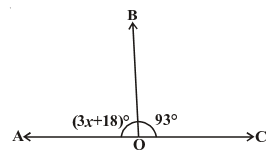ii.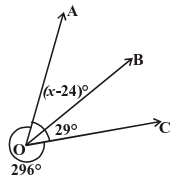iii.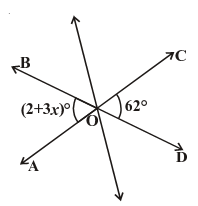iv.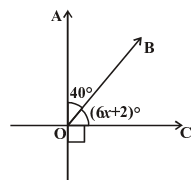Answer:(i) From the given figure,3x + 18° + 93° = 180°⇒ 3x + 111° = 180°⇒ 3x = 180°-111°⇒ 3x = 69°⇒ x =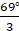∴ x = 24°(ii) From the given figure,(x-24)° + 29° + 296° = 360°⇒ (x-24)° = 360°-325°⇒ (x-24)° = 35°⇒ x = 35° + 24°∴ x = 59°(iii) From the given figure,(2 + 3x)° = 62°⇒ 3x = 62°-2° = 60°⇒ x =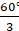∴ x = 20°(iv) From the given figure,40° + (6x + 2)° = 90°⇒ (6x + 2)° = 90°-40°⇒ (6x + 2)° = 50°⇒ 6x = 50°-2° = 48°⇒ x =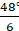∴ x = 8°Question 3.In the given figure lines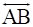and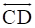intersect at O. If ∠AOC + ∠BOE = 70° and ∠BOD = 40°, find ∠BOE and reflex ∠COE.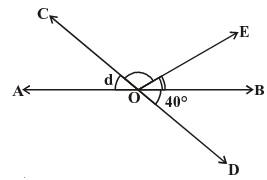Answer:Given that,The lines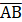and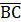intersect at O.∠AOC + ∠BOE = 70° ----(1)∠BOD = 40° ----(2)If the two lines intersect at a point then its vertically opposite angles are equal.∴ ∠AOC = ∠BODSubstitute (2) in (1)⇒ 40° + ∠BOE = 70°⇒∠BOE = 70°-40°∴∠BOE = 30°From the figure,AOB is a straight line and its angle is 180°So, ∠AOC + ∠BOE + ∠COE = 180°From equation (1)⇒ 70° + ∠COE = 180°⇒∠COE = 180°-70°∴∠COE = 110°Reflex ∠COE = 360° - 110° = 250°∴∠BOE = 30° and Reflex ∠COE = 250°Question 4.In the given figure lines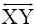and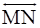intersect at O. If ∠POY = 90° and a: b = 2 : 3, find c.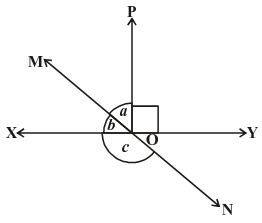Answer:Given that,The lines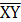and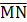intersect at O.From the figure, XOY is a straight line and its angle is 180°So, ∠XOM + ∠MOP + ∠POY = 180°From the given, Let ∠a = 2x and ∠b = 3x⇒∠b + ∠a + ∠POY = 180°----(1)Given that ∠POY = 90°Substitute the values in equation (1),⇒2x + 3x + 90° = 180°⇒5x + 90° = 180°⇒5x = 180°-90° = 90°⇒x =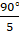∴ x = 18°⇒ ∠a = 2x = 2×18° = 36°⇒ ∠b = 3x = 3×18° = 54°From the figure, MON is a straight line and its angle is 180°⇒∠b + ∠c = 180°⇒54° + ∠c = 180°⇒∠c = 180°-54°∴ ∠c = 126°Question 5.In the given figure ∠PQR = ∠PRQ, then prove that ∠PQS = ∠PRT.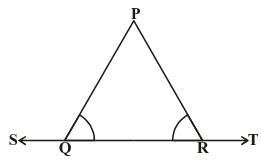Answer:In the figure, ST is a straight line and its angle is 180°So, ∠PQS + ∠PQR = 180° ----(1)And ∠PRT + ∠PRQ = 180°-----(2)From the two equations, we get∠PQS + ∠PQR = ∠PRT + ∠PRQGiven that,∠PQR = ∠PRQ⇒ ∠PQS + ∠PRQ = ∠PRT + ∠PRQ⇒ ∠PQS = ∠PRT + ∠PRQ -∠PRQ⇒ ∠PQS = ∠PRTSo, ∠PQS = ∠PRT is proved.Question 6.In the given figure, if x + y = w + z, then prove that AOB is a line.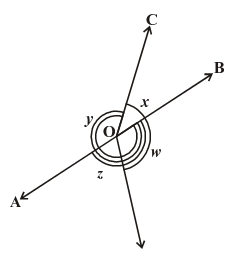Answer:In a circle, the sum of all angles is 360°∴ ∠AOC + ∠BOC + ∠DOB + ∠AOD = 360°⇒ x + y + w + z = 360°Given that, x + y = w + z⇒ w + z + w + z = 360°⇒ 2w + 2z = 360°⇒ 2(w + z) = 360°⇒ w + z = 180° or ∠DOB + ∠AOD = 180°If the sum of two adjacent angles is 180° then it forms a line.So AOB is a line.Question 7.In the given figure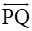is a line. Ray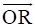is perpendicular to line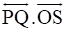is another ray lying between raysand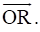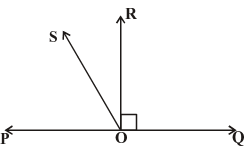Prove that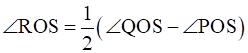Answer:Given that,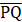is a line and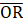is perpendicular to line.⇒ ∠POR = 90°The sum of linear pair is always equal to 180°∴ ∠POS + ∠ROS + ∠POR = 180°Substitute ∠POR = 90°⇒90° + ∠POS + ∠ROS = 180°⇒∠POS + ∠ROS = 90°∴ ∠ROS = 90°-∠POS----(1)⇒ ∠QOR = 90°Given that OS is another ray lying between OP and OR⇒∠QOS-∠ROS = 90°∴∠ROS = ∠QOS-90°----(2)On adding two equations (1) and (2) we get2∠ROS = ∠QOS-∠POS⇒ ∠ROS =(∠QOS-∠POS)So, ∠ROS =(∠QOS-∠POS) is proved.Question 8.It is given that ∠XYZ = 64° and XY is produced to point P. A ray YQ bisects ∠ZYP. Draw a figure from the given information. Find ∠XYQ and reflex ∠QYP.Answer:Let us draw a figure from the given,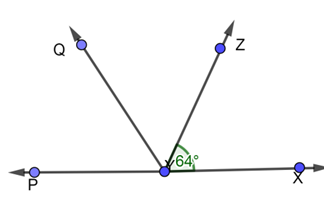Given that, a ray YQ bisects ∠ZYPSo, ∠QYP = ∠ZYQHere, PX is a straight line, so the sum of the angles is equal to 180°∠XYZ + ∠ZYQ + ∠QYP = 180°Given that, ∠XYZ = 64° and ∠QYP = ∠ZYQ⇒ 64° + 2∠QYP = 180°⇒ 2∠QYP = 180°-64° = 116°∴ ∠QYP =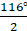= 58°Also, ∠QYP = ∠ZYQ = 58°Using the angle of reflection,∠QYP = 360°-58° = 302°∠XYQ = ∠XYZ + ∠ZYQ⇒∠XYQ = 64° + 58° = 122°∴ Reflex ∠QYP = 302° and ∠XYQ = 122°

PDF FILE TO YOUR EMAIL IMMEDIATELY PURCHASE NOTES & PAPER SOLUTION. @ Rs. 50/- each (GST extra)

HINDI ENTIRE PAPER SOLUTION

MARATHI PAPER SOLUTION

SSC MATHS I PAPER SOLUTION

SSC MATHS II PAPER SOLUTION

SSC SCIENCE I PAPER SOLUTION

SSC SCIENCE II PAPER SOLUTION

SSC ENGLISH PAPER SOLUTION

SSC & HSC ENGLISH WRITING SKILL

HSC ACCOUNTS NOTES

HSC OCM NOTES

HSC ECONOMICS NOTES

HSC SECRETARIAL PRACTICE NOTES

# 2019 Board Paper Solution

HSC ENGLISH SET A 2019 21st February, 2019

HSC ENGLISH SET B 2019 21st February, 2019

HSC ENGLISH SET C 2019 21st February, 2019

HSC ENGLISH SET D 2019 21st February, 2019

SECRETARIAL PRACTICE (S.P) 2019 25th February, 2019

HSC XII PHYSICS 2019 25th February, 2019

CHEMISTRY XII HSC SOLUTION 27th, February, 2019

OCM PAPER SOLUTION 2019 27th, February, 2019

HSC MATHS PAPER SOLUTION COMMERCE, 2nd March, 2019

HSC MATHS PAPER SOLUTION SCIENCE 2nd, March, 2019

SSC ENGLISH STD 10 5TH MARCH, 2019.

HSC XII ACCOUNTS 2019 6th March, 2019

HSC XII BIOLOGY 2019 6TH March, 2019

HSC XII ECONOMICS 9Th March 2019

SSC Maths I March 2019 Solution 10th Standard11th, March, 2019

SSC MATHS II MARCH 2019 SOLUTION 10TH STD.13th March, 2019

SSC SCIENCE I MARCH 2019 SOLUTION 10TH STD. 15th March, 2019.

SSC SCIENCE II MARCH 2019 SOLUTION 10TH STD. 18th March, 2019.

SSC SOCIAL SCIENCE I MARCH 2019 SOLUTION20th March, 2019

SSC SOCIAL SCIENCE II MARCH 2019 SOLUTION, 22nd March, 2019

XII CBSE - BOARD - MARCH - 2019 ENGLISH - QP + SOLUTIONS, 2nd March, 2019

# HSCMaharashtraBoardPapers2020

(Std 12th English Medium)

HSC ECONOMICS MARCH 2020

HSC OCM MARCH 2020

HSC ACCOUNTS MARCH 2020

HSC S.P. MARCH 2020

HSC ENGLISH MARCH 2020

HSC HINDI MARCH 2020

HSC MARATHI MARCH 2020

HSC MATHS MARCH 2020

# SSCMaharashtraBoardPapers2020

(Std 10th English Medium)

English MARCH 2020

HindI MARCH 2020

Hindi (Composite) MARCH 2020

Marathi MARCH 2020

Mathematics (Paper 1) MARCH 2020

Mathematics (Paper 2) MARCH 2020

Sanskrit MARCH 2020

Sanskrit (Composite) MARCH 2020

Science (Paper 1) MARCH 2020

Science (Paper 2)

Geography Model Set 1 2020-2021

MUST REMEMBER THINGS on the day of Exam

Are you prepared? for English Grammar in Board Exam.

Paper Presentation In Board Exam

How to Score Good Marks in SSC Board Exams

Tips To Score More Than 90% Marks In 12th Board Exam

How to write English exams?

How to prepare for board exam when less time is left

How to memorise what you learn for board exam

No. 1 Simple Hack, you can try out, in preparing for Board Exam

How to Study for CBSE Class 10 Board Exams Subject Wise Tips?

JEE Main 2020 Registration Process – Exam Pattern & Important Dates

NEET UG 2020 Registration Process Exam Pattern & Important Dates

How can One Prepare for two Competitive Exams at the same time?

8 Proven Tips to Handle Anxiety before Exams!